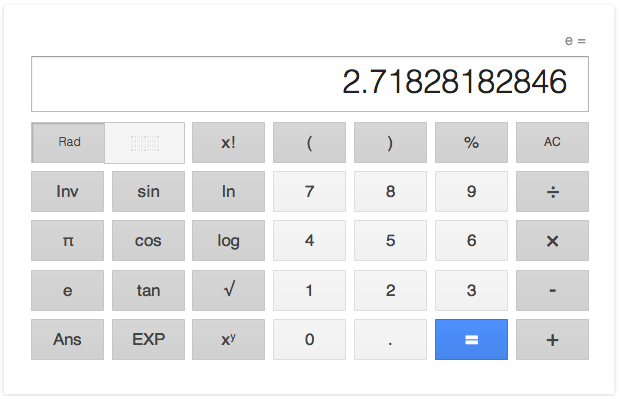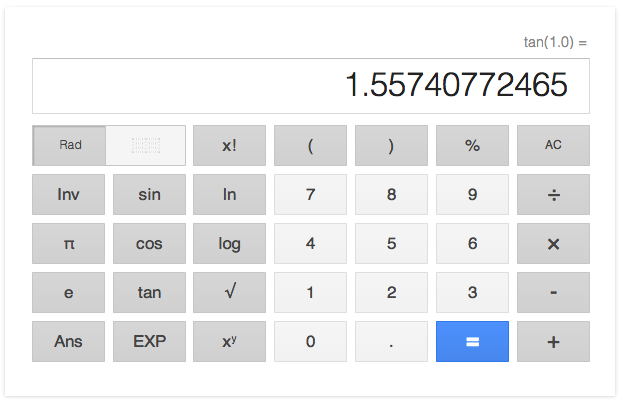## [SICP 1.3.3] Exercise 1.37-1.39 Memo

Exercise 1.37(a)- define k-term finite continued fraction.

Linear recursive version:

```
(define (cont-frac n d k)
(define (cont-frac-iter i)
(if (= i k)
(/ (n i) (d i))
(/ (n i) (+ (d i) (cont-frac-iter (+ i 1.0)))))
)
(cont-frac-iter 1)
)
```

Then how can we check if the number of k is enough for the calculation in the accuracy of 0.0001? We may be able to check it manually, but creating a checking function seems to be better for me.

```
(define (check-accuracy)
(define (iter k)
(if (< (abs (- (calc-ratio k) (/ 1 1.6180339887)) ) 0.0001)
k
(iter (+ k 1))
)
)
(iter 1)
)

```

And we get 10 iterations to get the accuracy in 0.0001.

In this case, the function passes calc-ratio method without using arguments. You can build an abstraction for checking arbitrary accuracy of arbitrary function if you prefer.

```
(define (check-accuracy accuracy f target)
(define (iter k)
(if (< (abs (- (f k) target) ) accuracy)
k
(iter (+ k 1))
)
)
(iter 1)
)

```

Exercise 1.37(b) Write linear-iterative version of cont-frac function ( if you’ve created the linear-iterative one in the Exercise 1.37(a), then create linear-recursive version )

The linear-recursive version processes the calculation from 1 to n, but we need to change this process from n to 1 for creating the linear-iterative one, because if we try to start from 1 and make it increment the number, the requirement of the result of (i + 1)th process occurs to determine the result of i-th process. Then the function will be as follows:

```
(define (cont-frac-linear-iterative n d k)
(define (cont-frac-iter numerator i)
(cond ((= i 1) numerator)
((= i k) (cont-frac-iter (/ (n (- i 1.0)) (+ (/ (n i) (d i)) (d (- i 1.0)))) (- i 1.0)))
(else (cont-frac-iter (/ (n (- i 1.0)) (+ numerator (d (- i 1.0)))) (- i 1.0)))
)
)
(cont-frac-iter 0 k)
)

```

Let’s check two versions of cont-frac function.

```
(define (calc-ratio k) (cont-frac (lambda (x) 1.0) (lambda (x) 1.0) k))
(define (calc-ratio2 k) (cont-frac-linear-iterative (lambda (x) 1.0) (lambda (x) 1.0) k))

(calc-ratio 100) ; 0.6180339887498948
(calc-ratio2 100) ; 0.6180339887498948

(calc-ratio 10000000) ; used up the memory
(calc-ratio2 10000000) ; 0.6180339887498948

```

Exercise 1.38 – define the natual logarithm e.

We finally get the expression of the 1, 2, 1, 1, 4, 1, 1, 6, 1, 1, 8, … as follows:

```
(define (e)
(+ 2 (cont-frac-linear-iterative
(lambda (a) 1.0)
(lambda (a) (if (< (remainder a 3) 2) 1.0 (* (/ 2.0 3.0) (+ a 1))))
1000
))
)

```

The output is:

```
(e) ; 2.7182818284590455

```(if (< (remainder a 3) 2) 1.0 [calculation] ) means that the element that (= (remainder a 3) 0) or (= (remainder a 3) 1) will be 1.0.

To get the meaning of [calculation] ( that is, (* (/ 2.0 3.0) (+ a 1)) ), we need some conversion of numbers.

n = 2, 5, 8, 11, 14

m = 1, 2, 3, 4, 5

a(m) = 2, 4, 6, 8, 10 = (* 2 m)

then m = (* (/ 1 3 ) ( n + 1 ))

so a(n) = (* 2 m) = (* 2 (* (/ 1 3 ) ( n + 1 ))) = (* (/ 2 3 ) ( n + 1 ))

Exercise 1.39 - Define a procedure (tan-cf x k) that computes an approximation to the tangent
function based on Lambert’s formula.

Note that the operand of the calculation in numerators is minus.

```
(define (tan-cf x k)
(cont-frac-linear-iterative
(lambda (a) (if (= a 1) x (- (* x x))))
(lambda (a) (- (* 2 a) 1))
k
)
)

```

The output is:

```
(tan-cf 1.0 1000) ; 1.557407724654902

```<<

Top

>>